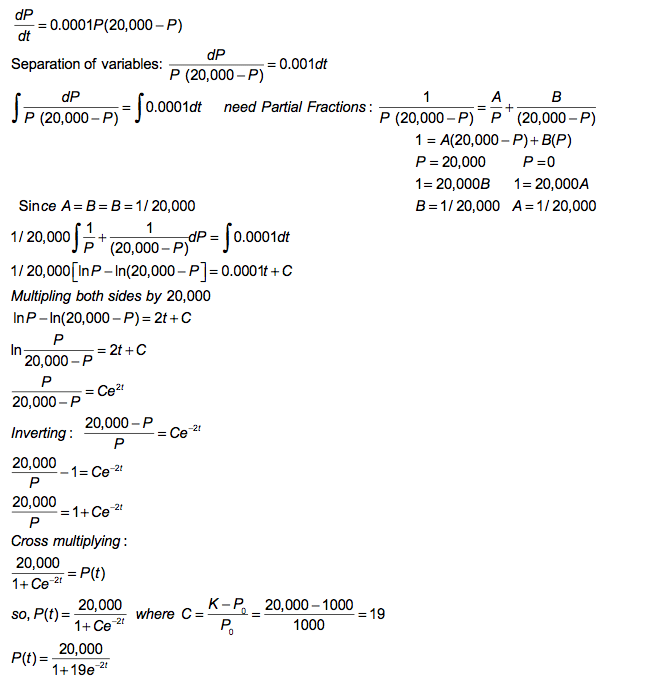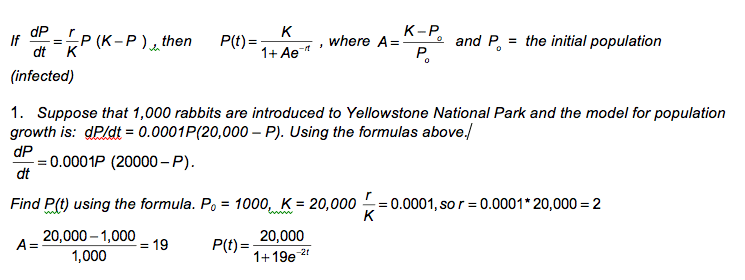## Logistic Differential Equation

Disease (Infection) and Population

The rate of change is proportional to the product of those infected (P), and those not infected (K – P) where K is the carrying capacity.  In other words, the rate of change is proportional to the product of the “Haves”  (P), and the “Have Nots” (K – P).#### Suppose that 1,000 rabbits are introduced to Yellowstone National Park and the model for population growth is: dP/dt = 0.0001P(20,000 – P). Find P(t) by integrating.#### Suppose that 1,000 rabbits are introduced to Yellowstone National Park and the model for population growth is: dP/dt = 0.0001P(20,000 – P). Find P(t) using formulas.#### Limits of dP/dt = 0.0001P(20,000 – P) and P(t)#### #28 from book: dP/dt = 0.0008P(700 – P) and P = 10 when t = 0. Find P(t) using formulas.